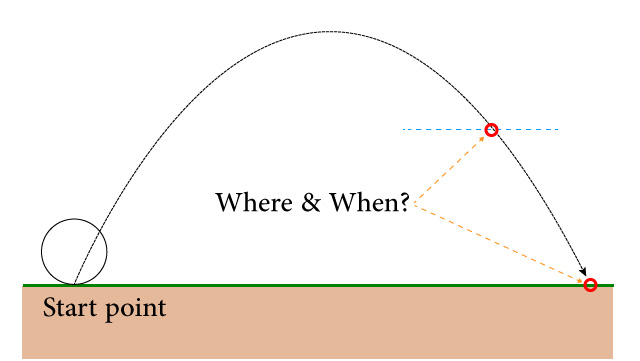# Where ball will drop?

Hello
I shoot a ball similar to the Angry Birds mechanics
For example, I want to know where the ball will land.
Or when it reaches to a specific point.
I used this very simple code to move the ball:

``````ball.transform.Translate (Vector3.forward * force * 2 * Time.deltaTime);
ball.transform.Translate (Vector3.up * (gravity / 5f) * Time.deltaTime);
``````So it looks like you’re not actually launching the ball, just moving it (with transform.Translate). To actually launch the ball, you could do something like `ball.getComponent<Rigidbody>().AddForce(new Vector3(forwardForce*Time.deltaTime, upwardsForce*Time.deltaTime, 0))`. If you have the ball’s current position and velocity and the force of gravity, you’ll be able to solve for where the ball will be at any time, and where it will land using some algebra. This is of course assuming that you aren’t using drag on the rigidbody — if so, you’d have to use differential equations which are quite a bit harder to use.

Say we have the ball at a point (3, 6, 0)m with a velocity of (2, 1, 0)m/s. Gravity is -9.8 m/s^2 in the Y-axis, which can be expressed as a vector of the form <0, -9.8, 0>
We can model the acceleration of the ball with the equation

a(t) = <0, -9.8, 0>

To get velocity, we take the indefinite integral (antiderivative), which gives us

v(t) = <0, -9.8, 0>*t + C

In this case, C is a vector representing the velocity of the ball. Taking the derivative of something only preserves how that thing changes over time, not its state when you took the derivative. Because of this, you have to specify a starting velocity vector otherwise it starts at zero. Since our velocity is (2, 1, 0) m/s, C = <2, 1, 0>

v(t) = <0, -9.8, 0>t + <2, 1, 0>

Now we integrate again to get the equation for position:

p(t) = <0, -9.8, 0>/2 * t^2 + <2, 1, 0>t + C

In this case, C is our current position (3, 6, 0)

p(t) = <0, -4.9, 0>t^2 + <2, 1, 0>t + <3, 6, 0>

So voila! We can find the position of the ball at any time using this equation. Of course, it ignores any collisions or external forces that might be exerted on the ball. Additionally, the point where you construct the equation (<3, 6, 0>) is t=0.

For the record, you can destruct the p(t) vector equation into three parametrics:

x(t) = 2t + 3
y(t) = -4.9t^2 + t + 6
z(t) = 0

Solve for the t-value that makes y(t) == 0, and you’ll know the time in the future that the ball will reach the ground. Same goes for any y or x value.

Overall, the above equations can be generalized as
constantAcceleration * (x^2)/2 + initialVelocity * x + initialPosition